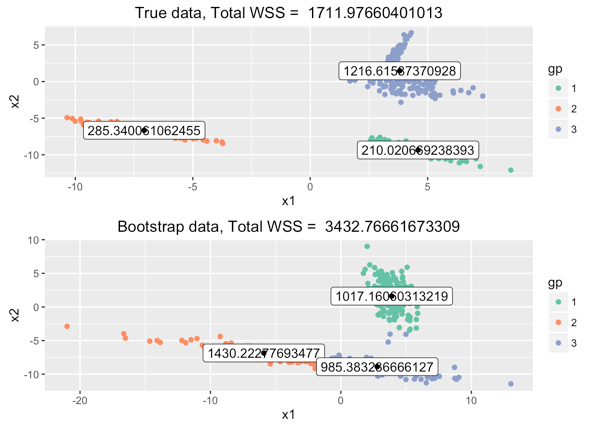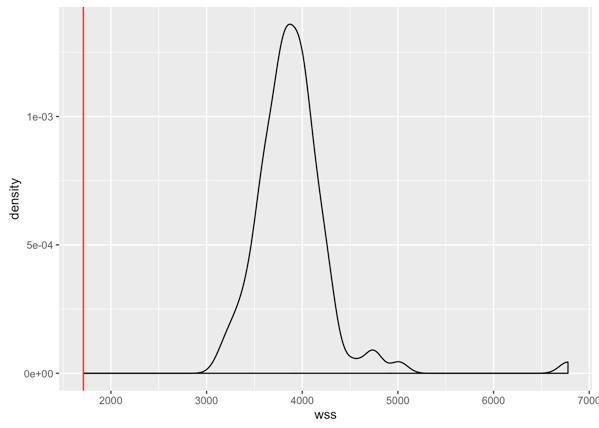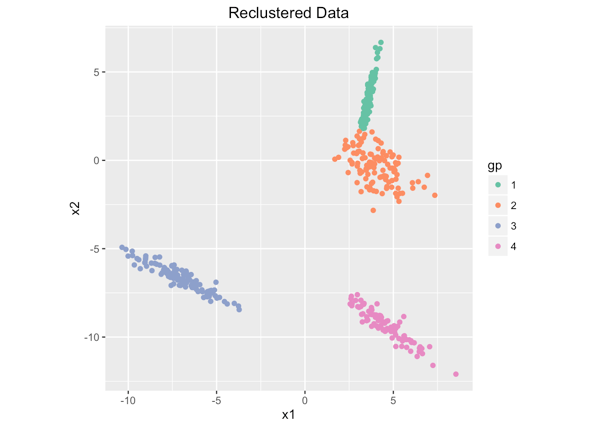# Finding the K in K-means by Parametric Bootstrap

One of the trickier tasks in clustering is determining the appropriate number of clusters. Domain-specific knowledge is always best, when you have it, but there are a number of heuristics for getting at the likely number of clusters in your data. We cover a few of them in Chapter 8 (available as a free sample chapter) of our book Practical Data Science with R.

We also came upon another cool approach, in the `mixtools` package for mixture model analysis. As with clustering, if you want to fit a mixture model (say, a mixture of gaussians) to your data, it helps to know how many components are in your mixture. The `boot.comp` function estimates the number of components (let’s call it k) by incrementally testing the hypothesis that there are k+1 components against the null hypothesis that there are k components, via parametric bootstrap.

You can use a similar idea to estimate the number of clusters in a clustering problem, if you make a few assumptions about the shape of the clusters. This approach is only heuristic, and more ad-hoc in the clustering situation than it is in mixture modeling. Still, it’s another approach to add to your toolkit, and estimating the number of clusters via a variety of different heuristics isn’t a bad idea.

### The Idea

Suppose this is our data:In two dimensions, it’s pretty easy to see how many clusters to try for, but in higher dimensions this gets more difficult. Let’s set as our null hypothesis that this data is broken into two clusters.We can now estimate the mean and covariance matrices of these two clusters, for instance by using principle components analysis. If we assume that the clusters were generated by gaussian processes with the observed means and covariance matrices, then we can generate synthetic data sets, of the same size as our real data,that we know have only two clusters, and also have the same means and covariances.This doesn’t look very much like our real data, but we don’t know that.

In other words, the full null hypothesis is that the data is composed of two gaussian clusters, of the observed mean and covariance.

Now we want to test the hypothesis that a data set is composed of three clusters (or more) against the null hypothesis that it has only two clusters. To do this, we generate several synthetic data sets as described above, and cluster them into three clusters. We evaluate each clustering by some measure of clustering goodness; a common measure is the total within-sum-of-squares (total WSS) (see also Chapter 8 from our book). We then compare the total WSS from three-clustering the real data with the distribution of total WSS of the synthetic data sets.

For a data set with two actual gaussian clusters, we would expect the total WSS of a three clustering to be lower than that of a two clustering, because total WSS tends to decrease as we partition the data into more (and internally “tighter”) clusters. Our simulations give us a plausible distribution of the range of total WSS that we would tend to see.

If the real data is really in two (gaussian) clusters, then when we three-cluster it, we would expect a total WSS within the range of our simulations. The hope is that if the data is actually in more than two clusters, then the clustering algorithm — we used K-means for our experiments — will discover a three-clustering that is *better* (lower total WSS) than what we saw in our simulations, because the data is grouped into tighter clusters than what our null hypothesis assumed. This is what happens in our example case. Here’s a comparison of the real data to the above simulated data set when both are three-clustered.Here’s a comparison of the total WSS of the three-clustered real data (in red) compared to 100 bootstrap simulations:Judging by these results, we can reject the null hypothesis that the data is in two clusters, and operate on the assumption that there are three or more clusters. In general, if the total WSS of the true data’s k+1 clustering is lower than at least 95% of the total WSS from the appropriate bootstrap simulations (for a significance level of p < 0.05), then we reject the null hypothesis that there are k clusters, and assume that the data has at least k+1 clusters. This continues with increasing values of k until we can no longer reject our null hypothesis. For this example, we found four clusters — which is in fact how we generated the test data.### Some Points to Consider

• Obviously, this method only makes sense if we believe that the clusters are “gaussian-like”. In the experiments we ran (there is a link to the code, below), we generated each cluster from an n-dimensional spherical gaussian to which we applied a random linear transformation and a random shift.
• The procedure we outlined above stops when we fail to reject the null hypothesis (`boot.comp` does, too). Sometimes the procedure gets “stuck,” whereby it fails to reject the null hypothesis at k, but then does reject it at k+1. So alternatively, we can run the procedure for a fixed set of k (say, in the range from 1 to 10), and then take as the number of clusters the last k where we fail to reject the null hypothesis (skipping over the “stuck points”). This sometimes “unsticks” the procedure — and sometimes it doesn’t, so we default to the “immediate stop” procedure in our interactive demonstration of this method (see link below).
• Even when the clusters in the data are truly generated from gaussians and we know k, K-means cannot always generate the same component parameters as a proper mixture model: K-means produces a “hard” clustering, or a partitioning where every point belongs to only one cluster, whereas a mixture model is a “soft” partitioning, where a point can belong probabilistically to multiple components. Specifically, K-means cannot recover a cluster that is contained within another cluster, or discriminate clusters that substantially overlap. A mixture model may be able to detect both these cases. So if you strongly believe that your data is a mixture of gaussians (or some other distribution), and you want to recover the population parameters rather than simply find a “best partitioning” of the data, you should use `mixtools` or another mixture model analysis package.
• We used a simple method-of-moments style approach to estimate the parameters of our gaussians. The `boot.clust` function uses maximum likelihood, which generally estimates the true parameters better (assuming the data is truly gaussian), and is unbiased.
• This idea should extend to other cluster metrics, like the Calinski-Harabasz Index, as well as to other clustering algorithms. We used K-means because it’s simple and fast. You can even modify the null hypothesis to cover other models of generating the clusters. Feel free to try your own experiments.

The code to generate the data sets in our experiments and run the parametric bootstrap is available at Github here. You can experiment with different dimensionalities of data and different numbers of clusters by altering the parameters in the R Markdown file `kcomp.Rmd`, but it is easier to play around by using the Shiny app we wrote to demonstrate the method. You can download the data generated by the Shiny app for your own experiments, as well.### Nina Zumel

Data scientist with Win Vector LLC. I also dance, read ghost stories and folklore, and sometimes blog about it all.